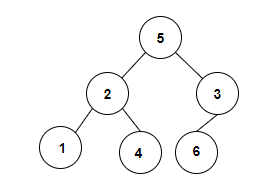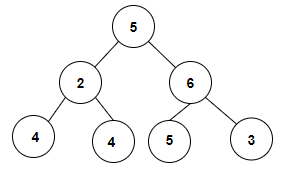# Count pairs in a binary tree whose sum is equal to a given value x in C++

We are given an integer value and a variable x and the task is to construct the binary tree and find the pairs having sum equals to the given value x.

For Example

## Input

int x = 5, The tree which will be created after inputting the values is given below −## Output

Count of pairs in a binary tree whose sum is equal to a given value x are: 2

## Explanation

we are given with an array of integer values that is used to form a binary
tree and we will check whether there is a pair present in a binary tree whose sum
equals to the given value x which is 5. So, the pairs formed are (2, 3) and (1, 4).

## Input

int x = 8, The tree which will be created after inputting the values is given below −## Output

Count of pairs in a binary tree whose sum is equal to a given value x are: 3

## Explanation

we are given with an array of integer values that is used to form a binary
tree and we will check whether there is a pair present in a binary tree whose sum
equals to the given value x which is 8. So, the pairs formed are (2, 6), (4, 4) and (5, 3).

Approach used in the below program is as follows

• Create a structure of a node that contains the data part and the left and right pointers which will be pointing to the left and right subtree.

• Input an integer value and use them to create a binary tree by entering the data to the node through left and right pointers.

• Input a value of x which will be used to calculate the pairs having sum value as of x.

• Create a boolean function to check whether the sum of pairs is x or not.

• Inside the function, check if root is NULL then return False

• Check IF root not equals ptr and data of root + data of ptr is equals to x then return True.

• Call check function recursively by passing the left pointer of root, ptr and value x and also right pointer of x, ptr and x. Now check whether any of the conditions is returning true then return true.

• Else, return false.

• Create a function total_pairs to calculate the count of pairs with sum as x

• Inside the function, check if ptr is NULL then return 0.

• Call the function check by passing root, ptr and x as an argument. IF the function returns true then increment the value of total pairs by 1

• Call function total_pairs recursively by passing root, left pointer of ptr, x and total and also pass root, right pointer of ptr, x and total.

• Print the result as an integer value stored in a variable total.

## Example

Live Demo

#include <bits/stdc++.h>
using namespace std;
struct tree_node {
int data;
tree_node *left, *right;
};
tree_node* create_node(int data){
tree_node* newNode = (tree_node*)malloc(sizeof(tree_node));
newNode−>data = data;
newNode−>left = newNode−>right = NULL;
}
bool check(tree_node* root, tree_node* ptr, int x){
if(root==NULL){
return false;
}
if (root != ptr && ((root−>data + ptr−>data) == x)){
return true;
}
if (check(root−>left, ptr, x) || check(root−>right, ptr, x)){
return true;
}
return false;
}
void total_pairs(tree_node* root, tree_node* ptr, int x, int& total){
if(ptr == NULL){
return;
}
if(check(root, ptr, x) == true){
total++;
}
total_pairs(root, ptr−>left, x, total);
total_pairs(root, ptr−>right, x, total);
}
int main(){
int x = 5;
int total = 0;
tree_node* root = create_node(5);
root−>left = create_node(2);
root−>right = create_node(3);
root−>left−>left = create_node(1);
root−>left−>right = create_node(4);
root−>right−>left = create_node(6);
total_pairs(root, root, x, total);
total = total / 2;
cout<<"Count of pairs in a binary tree whose sum is equal to a given value x are: "<< total;
return 0;
}

## Output

If we run the above code it will generate the following output −

Count of pairs in a binary tree whose sum is equal to a given value x are: 2